# KSEEB Solutions for Class 6 Maths Chapter 9 Data Handling Ex 9.1

Students can Download Chapter 9 Data Handling Ex 9.1 Questions and Answers, Notes Pdf, KSEEB Solutions for Class 6 Maths helps you to revise the complete Karnataka State Board Syllabus and score more marks in your examinations.

## Karnataka State Syllabus Class 6 Maths Chapter 9 Data Handling Ex 9.1

Question 1.
In a mathematics test, the following marks were obtained by 40 students, Arrange these marks in a table using tally marks.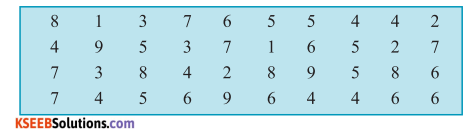a) Find how many students obtained marks equal to or more than 7.
b) How many students obtained marks below 4?
Solution:
By observing the marks scored by 40 students in the test we can construct the Table as follows :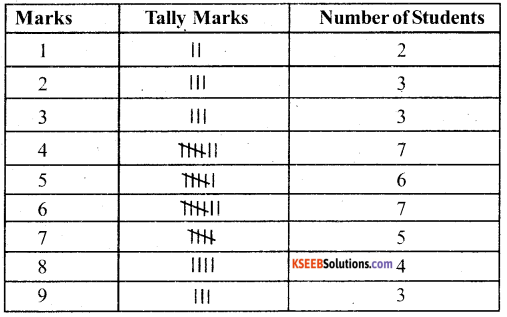a) The students who obtained their marks equal to or more than 7 are the students who obtained their marks as either of 7, 8 and 9. Hence number of these students = 5 + 4 + 3 = 12
b) The students who obtained their marks below 4 are the students who obtained their marks below 4 are the students who obtained their marks as either of 1, 2 and 3
Hence, number of these students = 2 + 3 + 3 = 8

Question 2.
Following is the choice of sweets of 30 students of class VI.
Laddoo, Barfi, Ladoo, Jalebi, Ladoo, Rasgulla, Jalebi,Ladoo, Barfi, Rasgulla, Ladoo, Jalbebi, Jalebi, Rasgulla, Ladoo, Rasgulla, Jalebi, Ladoo, Rasgulla, Ladoo, Ladoo, Barfi, Rasgulla, Rasgulla, Jalebi, Rasgulla, Ladoo, Rasgulla, Jalebi, Ladoo.
Solution:
By observing the choices the choices of sweets of 30 students, we can construct the table as bellow.
a) Arrange the names of sweets in a table using tally marks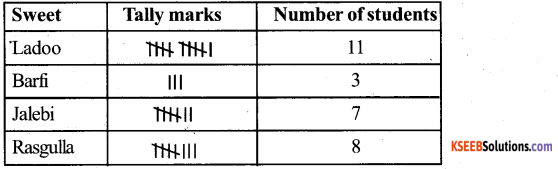b) Which sweet is preferred by most of the students?
Ladoo is the most perferred sweet as the most number of students (i,e, 11) prefer ladooQuestion 3.
Catherine threw a dice 40 times and noted the number appearing each time as shown below.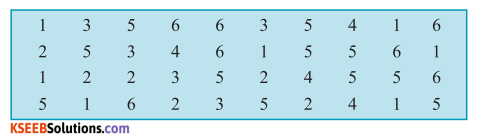Make a table and enter the data using tally marks. Find the number that appeared.
Solution: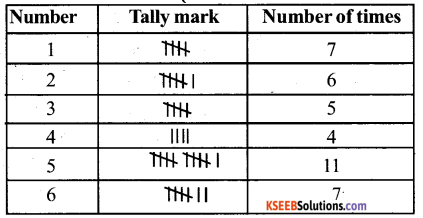a) The minimum number of times
The number which appeared the minimum number of times (i,e, 4 times) is 4

b) The maximum number of times
The number which appeared the maximum number of times (i,e, 11 times) is 5

c) Find those numbers that appear an equal number of times.
1 and 6 are the number which appears for the same number of times (i,e, 7 times)

Question 4.
Following pictograph shows the number of tractors in five villages.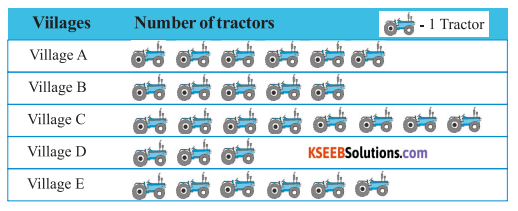Observe the pictograph and answer the following questions.
Solution:
i) Which village has the minimum number of tractors?
Village D has the minimum number of tractors, i.e. only 3 tractors

ii) Which village has the maximum number of tractors?
Village C has the maximum number of tractors i, e, 8 tractors.iii) How many more tractors village C has compared to village B.
Number of more tractors that village C has = 8 – 5 = 3

iv) What is the total number of tractors in all the five village?
Total number of tractors in all these villages = 6 + 5 + 8 + 3 + 6 = 28.

Question 5.
The number of girl students in each class of a co-educational middle school is depicted by the pictograph: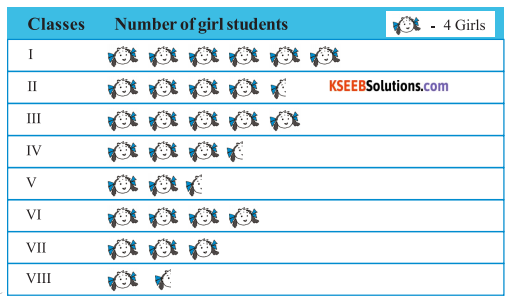Observe this pictograph and answer the following questions:
Solution:
From the above table, it can be concluded that in classes I, II, III, IV, V, VI, VII, VIII, there are 24, 18,20, 14, 10, 16, 12, 6 girls respectively.

a) Which class has the minimum number of girl student?
Class VIII has the minimum number of girls i.e. only 6 girls.

b) Is the number of girls in class VI less than the number of girls in Class V?
No, in class V and VI, there are 10 and 16 girls respectively clearly the number of girls is more in class VI than that in V

c) How many girls are there in class VII?
There are 12 girls in class VII.Question 6.
The sale of electric bulbs on different days of a week is shown below.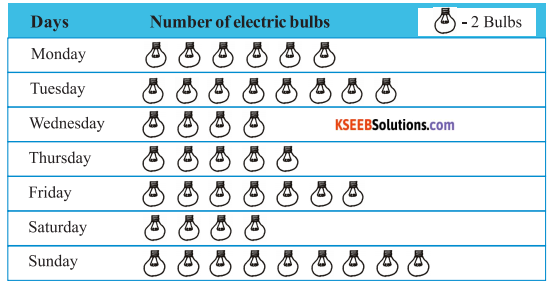Observe the pictograph and answer the following questions:
Solution:
a) How many bulbs were sold on friday?
14 bulbs were sold on friday.

b) On Which day were the maximum number of bulbs sold?
The maximum numbers of bulbs (i.e., 18) were sold on Sunday.

c) On Which of the days same number of bulbs were sold?
Equal numbers of bulbs (i.e., 8) were sold on Wednesday and Saturday.

d) On Which of the days minimum number of bulbs were sold?
The minimum numbers of bulbs (i.e., 8) were so Id on Wednesday and Saturday.

e) If one big carton can hold 9 bulbs. How many cartons were needed in the given week?
Total bulbs sold in the week = 12 + 16 + 8 + 10 + 14 + 8 + 18 = 86Question 7.
In a village six fruit merchants sold the following number of fruit baskets in a particular season: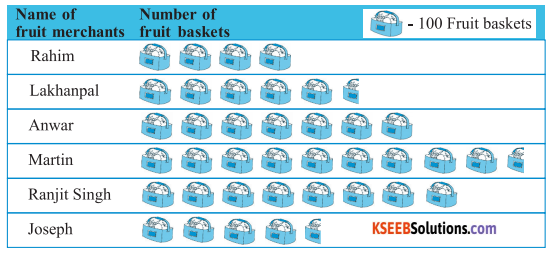Observe this pictograph and answer the following questions:
Solution:
a) Which merchant slid the maximum number of baskets?
b) How many fruit baskets were sold by answer?
c) The merchants who have sold 600 pr more number of baskets are planning to buy a godown for the next season, can you name them?
Solution:
From the above pictograph, it can be observed that the number of fruit basket sold by Rahim, Lakhanpai Anwar, Martin, Ranjit sigh, and Joseph are 400, 550, 700, 950, 800 and 450 respectively.
a) Martin sold the maximum number of baskets i.e, 950
b) Anwar sold 700 baskets.
c) Anwar, martin, and Ranjith singh are the 3 merchants who have sold more than 600 baskets.
Therefore they are planning to by a godown for the next season.